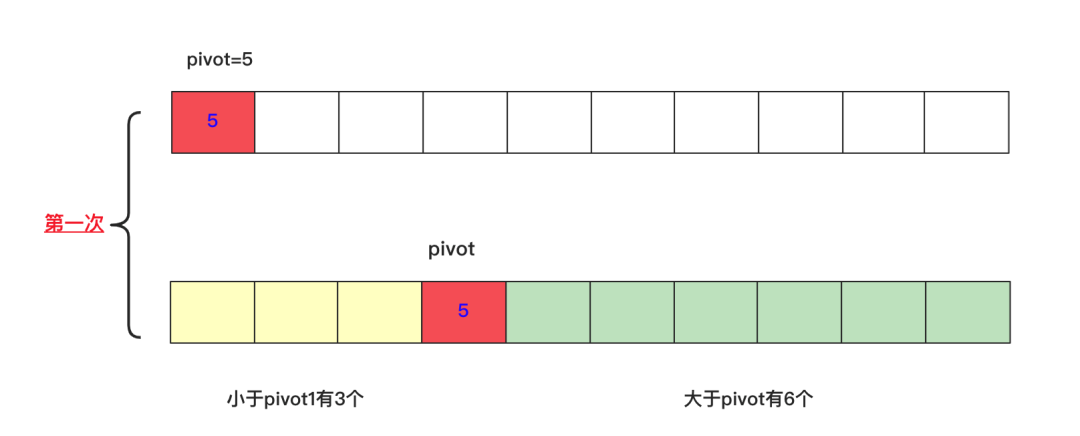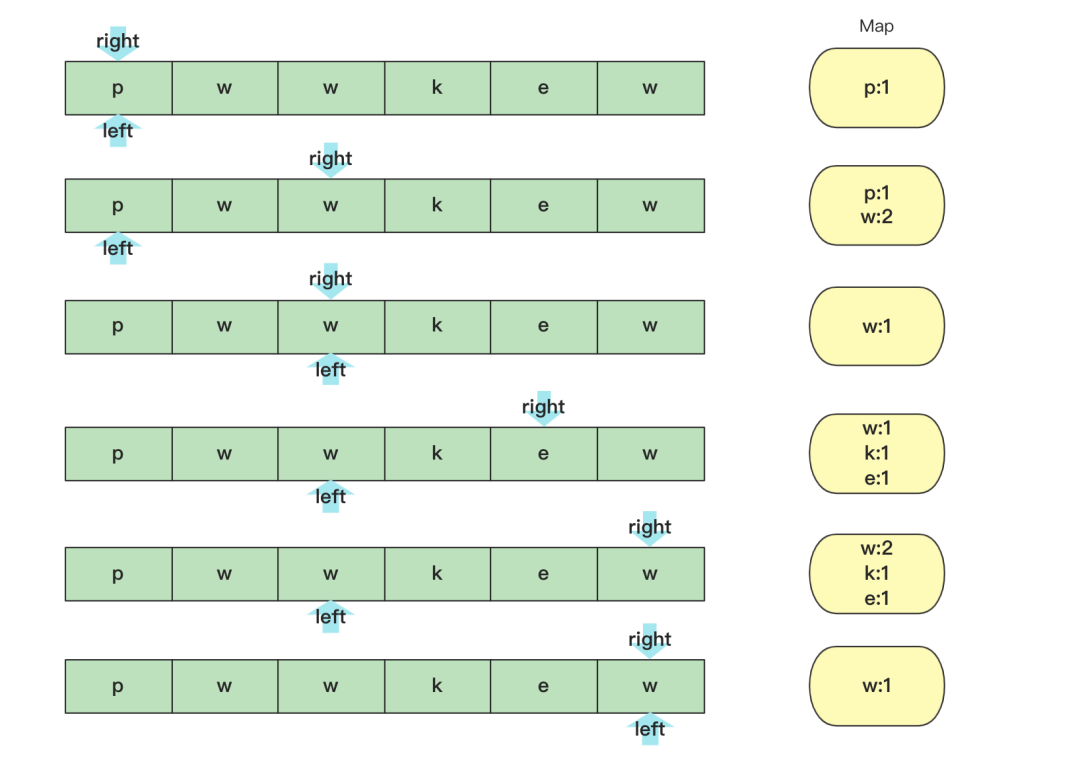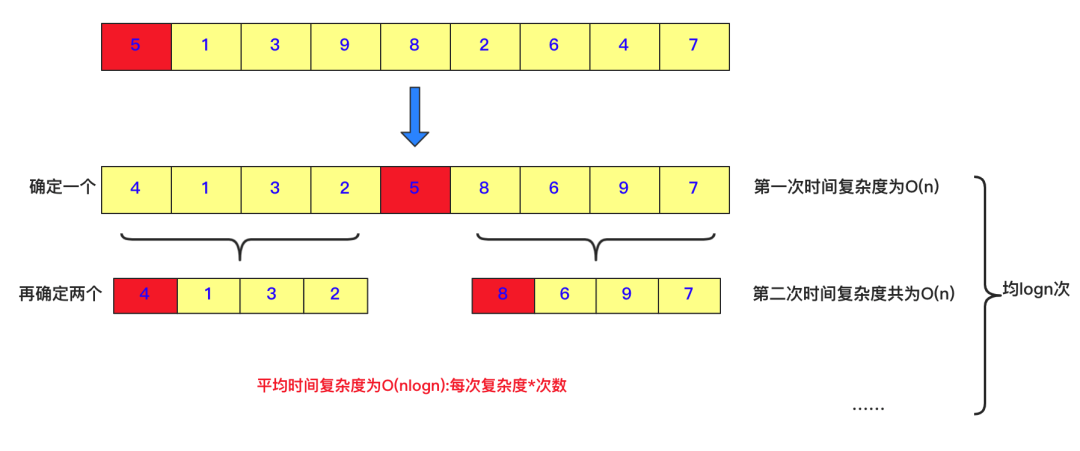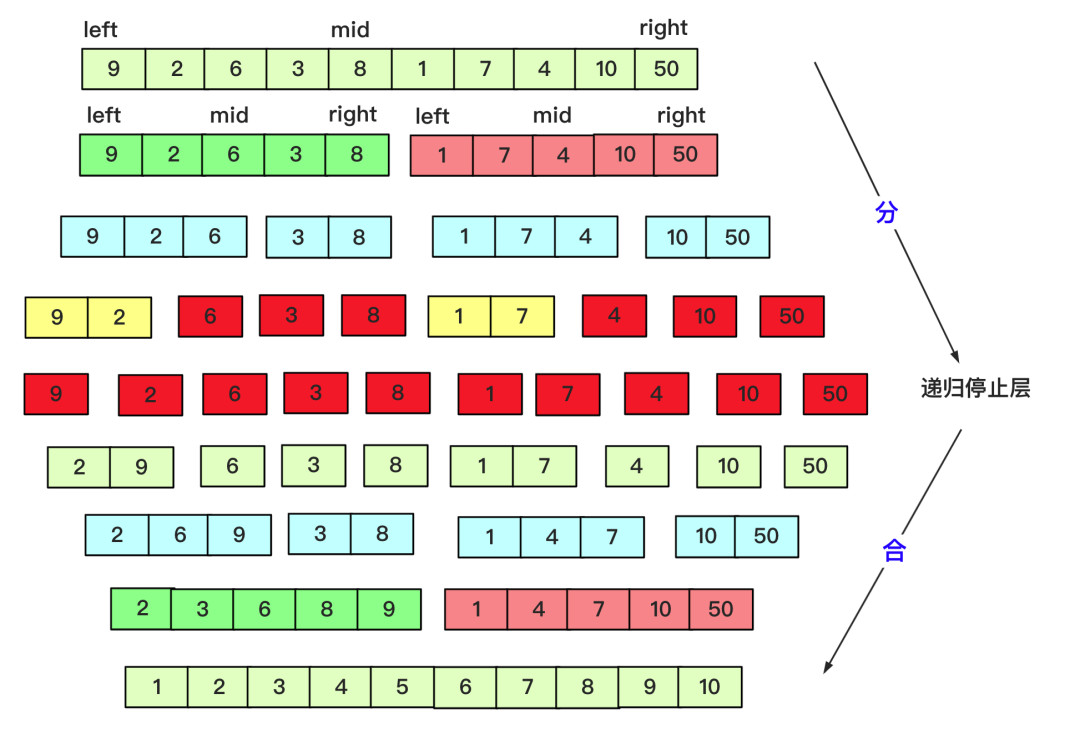# 必须干掉这10道，面试100%遇到！（四）

joytrian

0收藏

0X08 TOPK问题
TOPK问题真的非常经典，通常问的有最小的K个数，寻找第K大都是TOPK这种问题，这里就用力扣215寻找数组第K大元素作为板子。

TOPK的问题解决思路有很多，如果优化的冒泡或者简单选择排序，时间复杂度为O(nk),使用优化的堆排序为O(n+klogn)，不过掌握快排的变形就可以应付大体上的所有问题了(面试官要是让你手写堆排序那真是有点难为你了)。``````class Solution {
public int findKthLargest(int[] nums, int k) {
quickSort(nums,0,nums.length-1,k);
return nums[nums.length-k];
}
private void quickSort(int[] nums,int start,int end,int k) {
if(start>end)
return;
int left=start;
int right=end;
int number=nums[start];
while (left<right){
while (number<=nums[right]&&left<right){
right--;
}
nums[left]=nums[right];
while (number>=nums[left]&&left<right){
left++;
}
nums[right]=nums[left];
}
nums[left]=number;
int num=end-left+1;
if(num==k)//找到k就终止
return;
if(num>k){
quickSort(nums,left+1,end,k);
}else {
quickSort(nums,start,left-1,k-num);
}
}
}``````

0X09 无重复的最长子串(数组)``````class Solution {
public int lengthOfLongestSubstring(String s) {
int a[]=new int;
int max=0;//记录最大
int l=0;//left 用i 当成right，当有重复左就往右
for(int i=0;i<s.length();i++)
{
a[s.charAt(i)]++;
while (a[s.charAt(i)]>1) {
a[s.charAt(l++)]--;
}
if(i-l+1>max)
max=i-l+1;
}
return max;
}
}``````

0X10 排序``````public void quicksort(int [] a,int left,int right)
{
int low=left;
int high=right;
//下面两句的顺序一定不能混，否则会产生数组越界！！！very important！！！
if(low>high)//作为判断是否截止条件
return;
int k=a[low];//额外空间k，取最左侧的一个作为衡量，最后要求左侧都比它小，右侧都比它大。
while(low<high)//这一轮要求把左侧小于a[low],右侧大于a[low]。
{
while(low<high&&a[high]>=k)//右侧找到第一个小于k的停止
{
high--;
}
//这样就找到第一个比它小的了
a[low]=a[high];//放到low位置
while(low<high&&a[low]<=k)//在low往右找到第一个大于k的，放到右侧a[high]位置
{
low++;
}
a[high]=a[low];
}
a[low]=k;//赋值然后左右递归分治求之
quicksort(a, left, low-1);
quicksort(a, low+1, right);
}````````````private static void mergesort(int[] array, int left, int right) {
int mid=(left+right)/2;
if(left<right)
{
mergesort(array, left, mid);
mergesort(array, mid+1, right);
merge(array, left,mid, right);
}
}

private static void merge(int[] array, int l, int mid, int r) {
int lindex=l;int rindex=mid+1;
int team[]=new int[r-l+1];
int teamindex=0;
while (lindex<=mid&&rindex<=r) {//先左右比较合并
if(array[lindex]<=array[rindex])
{
team[teamindex++]=array[lindex++];
}
else {
team[teamindex++]=array[rindex++];
}
}
while(lindex<=mid)//当一个越界后剩余按序列添加即可
{
team[teamindex++]=array[lindex++];

}
while(rindex<=r)
{
team[teamindex++]=array[rindex++];
}
for(int i=0;i<teamindex;i++)
{
array[l+i]=team[i];
}
}``````